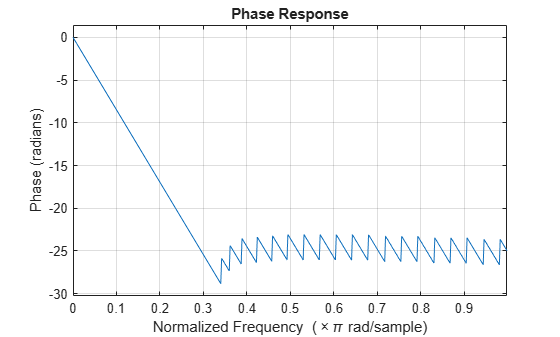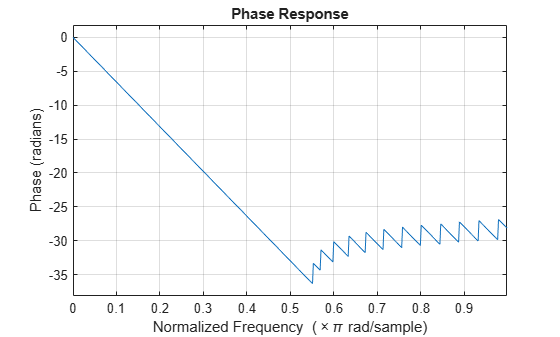# phasez

Phase response of digital filter

## Syntax

``[phi,w] = phasez(b,a,n)``
``[phi,w] = phasez(sos,n)``
``[phi,w] = phasez(d,n)``
``````[phi,w] = phasez(___,n,'whole')``````
``````[phi,f] = phasez(___,n,fs)``````
``phi = phasez(___,f,fs)``
``phi = phasez(___,w)``
``phasez(___)``

## Description

````[phi,w] = phasez(b,a,n)` returns the `n`-point phase response vector `phi` and the corresponding angular frequency vector `w` for the digital filter with the transfer function coefficients stored in `b` and `a`.```
````[phi,w] = phasez(sos,n)` returns the `n`-point phase response corresponding to the second-order sections matrix `sos`.```
````[phi,w] = phasez(d,n)` returns the `n`-point phase response for the digital filter `d`.```
``````[phi,w] = phasez(___,n,'whole')``` returns the phase response at `n` sample points around the entire unit circle. This syntax can include any combination of input arguments from the previous syntaxes.```
``````[phi,f] = phasez(___,n,fs)``` returns the frequency vector.```
````phi = phasez(___,f,fs)` returns the phase response vector `phi` evaluated at the physical frequencies supplied in `f`. This syntax can include any combination of input arguments from the previous syntaxes.```
````phi = phasez(___,w)` returns the unwrapped phase response in radians at frequencies specified in `w`.```

example

````phasez(___)` with no output arguments plots the phase response of the filter.```

## Examples

collapse all

Use `designfilt` to design an FIR filter of order 54, normalized cutoff frequency $0.3\pi$ rad/s, passband ripple 0.7 dB, and stopband attenuation 42 dB. Use the method of constrained least squares. Display the phase response of the filter.

```Nf = 54; Fc = 0.3; Ap = 0.7; As = 42; d = designfilt('lowpassfir','CutoffFrequency',Fc,'FilterOrder',Nf, ... 'PassbandRipple',Ap,'StopbandAttenuation',As, ... 'DesignMethod','cls'); phasez(d)```Design the same filter using `fircls1`. Keep in mind that `fircls1` uses linear units to measure the ripple and attenuation.

```pAp = 10^(Ap/40); Apl = (pAp-1)/(pAp+1); pAs = 10^(As/20); Asl = 1/pAs; b = fircls1(Nf,Fc,Apl,Asl); phasez(b)```Design a lowpass equiripple filter with normalized passband frequency $0.45\pi$ rad/s, normalized stopband frequency $0.55\pi$ rad/s, passband ripple 1 dB, and stopband attenuation 60 dB. Display the phase response of the filter.

```d = designfilt('lowpassfir', ... 'PassbandFrequency',0.45,'StopbandFrequency',0.55, ... 'PassbandRipple',1,'StopbandAttenuation',60, ... 'DesignMethod','equiripple'); phasez(d)```Design an elliptic lowpass IIR filter with normalized passband frequency $0.4\pi$ rad/s, normalized stopband frequency $0.5\pi$ rad/s, passband ripple 1 dB, and stopband attenuation 60 dB. Display the phase response of the filter.

```d = designfilt('lowpassiir', ... 'PassbandFrequency',0.4,'StopbandFrequency',0.5, ... 'PassbandRipple',1,'StopbandAttenuation',60, ... 'DesignMethod','ellip'); phasez(d)```## Input Arguments

collapse all

Transfer function coefficients, specified as vectors. Express the transfer function in terms of `b` and `a` as

`$H\left(z\right)=\frac{B\left(z\right)}{A\left(z\right)}=\frac{{b}_{1}+{b}_{2}{z}^{-1}\cdots +{b}_{n}{z}^{-\left(n-1\right)}+{b}_{n+1}{z}^{-n}}{{a}_{1}+{a}_{2}{z}^{-1}\cdots +{a}_{m}{z}^{-\left(m-1\right)}+{a}_{m+1}{z}^{-m}}$`

Example: `b = [1 3 3 1]/6` and `a = [3 0 1 0]/3` specify a third-order Butterworth filter with normalized 3 dB frequency 0.5π rad/sample.

Data Types: `double` | `single`
Complex Number Support: Yes

Number of evaluation points, specified as a positive integer scalar no less than 2. When `n` is absent, it defaults to 512. For best results, set `n` to a value greater than the filter order.

Second-order section coefficients, specified as a matrix. `sos` is a K-by-6 matrix, where the number of sections, K, must be greater than or equal to 2. If the number of sections is less than 2, the function treats the input as a numerator vector. Each row of `sos` corresponds to the coefficients of a second-order (biquad) filter. The ith row of `sos` corresponds to `[bi(1) bi(2) bi(3) ai(1) ai(2) ai(3)]`.

Example: `s = [2 4 2 6 0 2;3 3 0 6 0 0]` specifies a third-order Butterworth filter with normalized 3 dB frequency 0.5π rad/sample.

Data Types: `double` | `single`
Complex Number Support: Yes

Digital filter, specified as a `digitalFilter` object. Use `designfilt` to generate a digital filter based on frequency-response specifications.

Example: ```d = designfilt('lowpassiir','FilterOrder',3,'HalfPowerFrequency',0.5)``` specifies a third-order Butterworth filter with normalized 3 dB frequency 0.5π rad/sample.

Sample rate, specified as a positive scalar. When the unit of time is seconds, `fs` is expressed in hertz.

Data Types: `double`

Angular frequencies, specified as a vector and expressed in rad/sample. `w` must have at least two elements, because otherwise the function interprets it as `n`. `w` = π corresponds to the Nyquist frequency.

Frequencies, specified as a vector. `f` must have at least two elements, because otherwise the function interprets it as `n`. When the unit of time is seconds, `f` is expressed in hertz.

Data Types: `double`

## Output Arguments

collapse all

Phase response, returned as a vector. If you specify `n`, then `phi` has length `n`. If you do not specify `n`, or specify `n` as an empty vector, then `phi` has length 512.

If the input to `phasez` is single precision, the function computes the phase response using single-precision arithmetic. The output `phi` is single precision.

Angular frequencies, returned as a vector. `w` has values ranging from 0 to π. If you specify `'whole'` in your input, the values in `w` range from 0 to 2π. If you specify `n`, `w` has length `n`. If you do not specify `n`, or specify `n` as the empty vector, `w` has length 512.

Frequencies, returned as a vector expressed in hertz. `f` has values ranging from 0 to `fs`/2 Hz. If you specify `'whole'` in your input, the values in `f` range from 0 to `fs` Hz. If you specify `n`, `f` has length `n`. If you do not specify `n`, or specify `n` as an empty vector, `f` has length 512.

## Version History

Introduced before R2006a